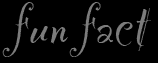# Let There Be Light, or... Heat?

### Purpose

The purposes of this lab are for students to determine which type of light bulb, compact fluorescent or incandescent, is more efficient at converting electrical energy to light instead of heat and for students to calculate the amount and cost of electricity used to power each type of light for 28 hours per week for a year.

### Key Science Topics

• Heat vs. temperature
• Power dissipated by a device

### Student Prior Knowledge

• Students should be able to calculate the power in Watts dissipated by a device when voltage and current are measured.
• Students should know how to calculate kilowatt-hours.
• Students should recognize that infrared radiation is heat.

### Materials

• Compact fluorescent light bulbs, with lamp base
• Incandescent Light bulbs, with lamp base
• Kil-A-Watt meter (see suggestions, below)

### Background

Students will examine whether a compact fluorescent light bulb or an incandescent light bulb produces more heat in two different activities. Students will hold a radiometer in front of each type of light bulb to observe which bulb makes the radiometer spin faster. Then, students will record data obtained from a Kil-A-Watt meter to determine which type of bulb uses more electricity.

Lowes rates light bulbs based on four hours of usage per day (www.lowes.com). Thus, the use per week is 28 hours. Students may comment that they use lights more or less often.  Encourage them to think about how often lights are left on in rooms no one is using. As used in the teacher answer key, the price of electricity in Ohio, as of this writing, is \$0.11 per kilowatt hour. Encourage your students to find out what is the local price for electricity prior to working on this activity.

Please remind students that their results are for only one light bulb. Encourage them to think about how many IL and CFL bulbs are in their house. Perhaps as an extension to this activity, you may want to have your students count each type of light bulb.

### Suggestions

Ideally, this activity is to be done with students visiting stations. I usually have six groups of three to four students. For this lab, each group gets their own radiometer and I have three lamp bases with a CFL plugged into a Kil-A-Watt meter and three lamp bases with an IL plugged into a Kil-A-Watt meter. Students start at one type of light bulb, and then switch to the other.

Kil-A-Watt meters are available for about \$30.00. If this is cost prohibitive, one Kil-A-Watt meter could be used by the teacher and the students could record the voltage and current data. Another possibility is to write to your local power company to see if they would donate Kil-A-Watt meters. If no Kil-O-Watt meter is available, feel free to provide the students with the sample voltage, current, and power data given in the Teacher Answer Key

I have students measure the power on the Kil-A-Watt meter.They can calculate the power from the voltage and current measured, but it will not be the same value because the light bulbs are non-Ohmic; in other words, the resistance of the light bulb changes as the filament increases in temperature.

Published: 26 January, 2022The density of the planet Saturn is less than the density of water. Find a bathtub big enough, and Saturn would float!